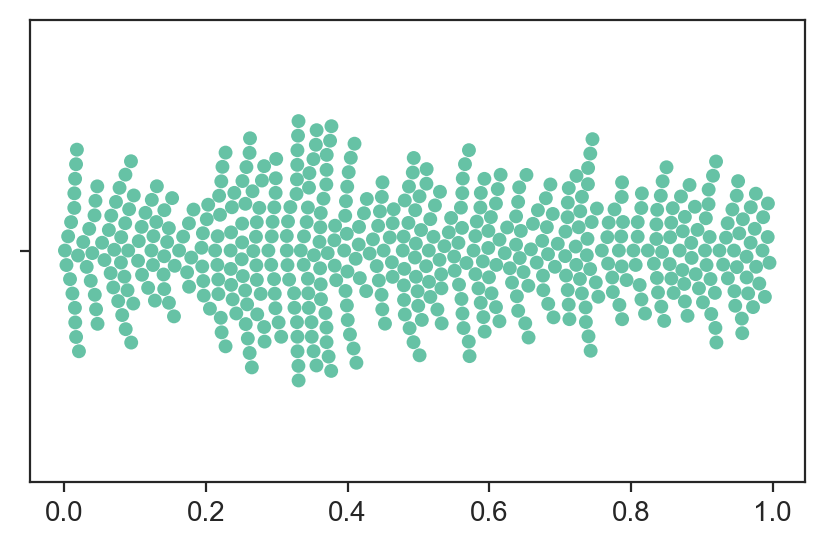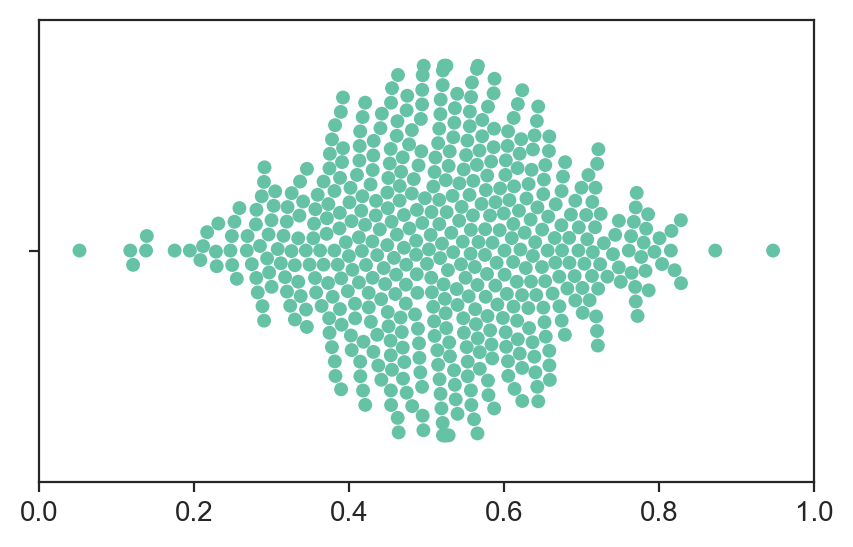[blog] | [about]

# Bob's Regression Riddle

Suppose that a friend ("Bob") comes to you with the following problem: he has a dataset with 1000 values, all between 0 and 1, and he wants to build a simplified "regression" model to predict the values in this dataset. It's "simplified" because there is no predictor variable, only the target attribute, which you take to mean that he basically wants a function that guesses each value in the dataset.

Bob doesn't know how the data was produced, but he knows that the mean is 0.5, and that the swarmplot is like this:To predict these values, he is considering three models:

1. A Mean Regressor that always predicts the mean of the data (0.5).
2. An Uniform Regressor, which predicts a value in $[0, 1]$ that is randomly sampled from an uniform distribution.
3. A Gaussian Regressor, which predicts a value in $[0, 1]$ that is randomly sampled from a normal distribution.

Which of these three models would you recommend to Bob, if his goal is to minimize mean squared error?

Now before we continue, let us consider a second scenario: Bob comes to you and reveals what he has just discovered: the dataset values were indeed sampled from an Uniform Distribution between 0 and 1.

Which model would you suggest for this new scenario?

Proceed.

A third and final scenario. What if the plot Bob showed you was instead like this:Which is the best model for this third scenario?

The best model is always the Mean Regressor. In all three cases, predicting the mean is the way to go, since it is the mathematical definition of the MSE's minimum. You literally cannot do better than the mean.

It might be tempting to try to match the model you're using to the data you have, but since we're trying to minimize the MSE, it is actually detrimental: if the data came from an Uniform distribution, the Uniform regressor actually has double the Mean Regressor's error. Here's the Python code, if you don't believe me:

y = np.random.uniform(0, 1, 1000000)
get_mse = lambda y_pred : np.sum((y - y_pred)**2) / len(y)

# Mean Regressor
print(get_mse([0.5] * len(y)))
>> 0.08329726707282648

# Uniform Regressor
print(get_mse(np.random.uniform(0, 1, len(y))))
>> 0.16678483232356572

# Gaussian Regressor
# (using parameters that generated the last figure)
print(get_mse(np.random.normal(0.5, 0.1, len(y))))
>> 0.09325200946333051


(You can also easily verify this mathematically.)

This question may seem like ML 101 to you, but I was surprised last week when I posed the same problem to many friends and found them all falling into the same trap: saying that the best model is the one that fits the data distribution. And that makes sense, doesn't it? If you know something about how the data was generated, you should use that to improve your model! But this would only be the case if we were trying to minimize something like KL Divergence, or if we were trying to create a model to generate more data. In this scenario, since we want to minimize the MSE, predicting the mean is the way to go (in the absence of better models, of course).

So moral of the story: Although you may sometimes discover important and actionable information about the problem you're trying to solve, it may not actually be relevant to what you're doing! In fact, using this information may actually leave you worse off!!

If you were unfazed by these questions, congratulations. Here's a picture of my cat.